AST 3010: Advanced Topics in Stellar
AST 3010: Advanced Topics in Stellar Astronomy (2011)
Transients

 Lectures Tuesdays 3 PM and Thursdays 1 PM in AB 113 (first class Th, Jan 13). Lecturer Marten van Kerkwijk, MP 1203B, 416-946-7288, mhvk@astro (utoronto.ca) Office hours TBD (likely after each class, or by appointment) Web page http://www.astro.utoronto.ca/~mhvk/AST3010/ notes pdf

## Synopsis

Transient stars sometimes appear on the sky. In this course, we will first discuss "transient physics," the physics we require to understand known types of transients - novae, supernovae, X-ray and γ-ray bursts - and their aftermath, comparing predicted behaviour with observations. Next, we will turn to "possible transients," systematically exploring what systems could lead to transients, discussing which ones might have possible observational counterparts, and determining how these could be found by the current and next generations of transients surveys. The goal is to produce a complete review of what one might expect, at a level that is useful for transient surveys, and ideally is suitable for publication (if so, by the class as a whole; surprisingly, such a review of expected types of transients does not yet seem to exist).

### Transient Physics

• Small Nuclear Runaways: Shell Flashes and Detonations
• Large Nuclear Runaways: He Flash, Carbon Deflagration.
• Sudden Removal of Pressure Support: Electron Capture, Core Collapse, Pair Instability.
• Mergers: Stars, White Dwarfs, and Neutron Stars
• Evolution of Ejecta: Expansion and Radioactive Heating
We'll start with an overview, then go through the evolution of low, intermediate, and high-mass stars, briefly discuss binary evolution, and end with a discussion of lightcurves.

### Possible Transients

We will explore systematically what types of transients one might expect from single stars, binaries, and clusters of stars. We will focus on binaries, checking for any sensible combination of two types of stars how they could interact during their evolution, and what final product one might expect. For a rough sense of the aim, see the outcome grid made by Stephen Justham (KIAA/Beijing).

## Course texts

Hopefully, there will be a course text by the end! In the meantime, we will have to do with review articles, etc. What will be important is to bring a good sense of the structure and evolution of stars and compact objects. Minimum pre-requisite is the (equivalent of the) undergraduate course AST 320; better, AST 1410 (offered this Fall); see that course web page for suggestions for books (personally, I learned most from Stellar Structure and Evolution, by Kippenhahn & Weigert [Springer-Verlag, 1990]; also good is Supernovae & nucleosynthesis, by Arnett [Princeton Univ. Press, 1996]).

## Evaluation

Problem sets and mini-research studies (60%), oral presentations (20%), oral exam (20%).

## Mini Research Projects

All students will investigate some particular types of systems (say, a ONe core white dwarf with a He core white dwarf companion), research what sort of transients these might produce, write up the results, and present them in class.
Practically, I hope to divide ourselves in three groups, on white dwarfs (accreting and merging), main-sequence/giant stars (including mergers with white dwarfs), and neutron stars/black holes (including mergers with other types). I would be part of all groups. A growing list of source combinations and links to references can be found at types.html.
For each specific topic, I'd hope to proceed as follows:
• Research and write up draft.
• Circulate among group for comments.
• Circulate among class and present (probably best on the board).
• Include further comments in final version.

## 1  Introduction

### What is a transient?

Two possible definitions of an "interesting" transient: (1) large ( > 100?) increase in brightness, lasts much less than human lifetime, and recurs infrequently; (2) A significant fraction ( > 1 %?) of the total energy available is used in a short time. One may also want to distinguish between disruption (one-off) and eruption (can repeat).

### Known eruptions, bursts, flashes, and flares

1. Failure of hydrostatic equilibrium (HE): Luminous Blue Variable (LBV) eruptions, AGB shells
2. Run-away fusion: shell flashes, Novae, X-ray bursts.
3. Magnetic reconnection: M star flares, soft gamma-ray repeaters (SGR).
4. Accretion instability: Dwarf novae, X-ray novae, FU Ori outbursts.
Known to occur but not yet(?) observed: helium flash.
We'll ignore magnetic bursts from here on.

### Known disruptions

1. Failure of HE: core-collapse SNe.
2. Run-away fusion: SN Ia.
3. Mergers: (some?) luminous red novae, short gamma-ray bursts (GRB)?
Known to occur but not yet(?) observed: mergers of different types of objects.
Predicted but not yet(?) observed: in single stars, pair-instability supernovae, carbon deflagration supernovae, electron-capture supernovae; in binaries, accretion-induced collapse of white dwarf to neutron star, accretion-induced collapse of neutron star to black hole (or quark star).

### Basic energetics

#### Gravitational

 E ∼ GM∆M R
(1.1)
For main-sequence stars ((M,R)=1 M,1 R), white dwarfs (1 M,0.01 R), and neutron stars (1.4 M,10 km), this corresponds to  ∼ 2×10−6, 2×10−4, and 2×10−1∆M c2.
Core-collapse supernovae and neutron-star mergers  :  ∼ 1053.5 erg.
Main-sequence mergers  :  ∼ 1048.5 erg.
Luminous Blue Variable   (M=50 M, ∆M=1 M, R=50 R):  ∼ 1048.5 erg.
Accretion burst   (∆M=· Mt ≅ 10−8M yr1×10−1 yr):  ∼ 1044.5 erg for NS,  ∼ 1041.5 for WD.

### Nuclear

 E ∼ ∆M mp Q,
(1.2)
where Q is the energy released per nucleon,  ∼ 7 MeV for fusing hydrogen to helium,  ∼ 1 MeV for other reactions. Thus, one gains  ∼ 7×10−3 and 1×10−3∆Mc2, respectively.
SN Ia  :  ∼ 1051.5 erg.
Novae  : One might think ∆M ≅ 10−4 M, hence E ≅ 1048 erg, but in reality much less is emitted:  ∼ LEdd×10−1 yr ≅ 1045 erg (radiation dominates the energetics). Material is ejected before it can be fused.
X-ray bursts   (∆M ≅ 10−9M yr1×104 s):  ∼ 1039 erg (now consistent with  ∼ L Edd×10 s; no mass leaves the neutron star).

## 2  Stability

### Dynamical stability

For a star to be stable to a density perturbation (upon, e.g., compression), pressure has to increase faster than gravity. For a polytrope, P ∝ ρΓ ∝ R−3Γ, with Γ = ([5/3],[4/3]) for the simplest non-relativistic and relativistic cases. For a star in hydrostatic equilibrium, P ∝ R−4. Hence, stability requires Γ ≥ [4/3] (for a star not described by a polytrope, a suitable average of Γ).
Ionisation in its most general form causes Γ to drop (the work compressing matter goes into ionisation rather than increasing the kinetic energy of the constituent particles). Examples: molecular dissociation, ionisation, pair creation, and nuclear dissociation. Capture of energetic electrons on protons (to form neutrons) has a similar effect.

### Gravothermal specific heat

For a star to be stable to a temperature perturbation, upon, e.g., an increase in fusion rate, the star has to expand and cool, i.e., have negative gravothermal specific heat (see KW, Ch. 25). For an ideal gas, the virial theorem shows this is the case; for a degenerate gas, though, Ekin (like pressure) does not depend on temperature.
Note that if cooling increases with increasing temperature (like for neutrino cooling, but unlike radiative losses), the situation reverses: this is unstable for an ideal gas, stable for a degenerate one.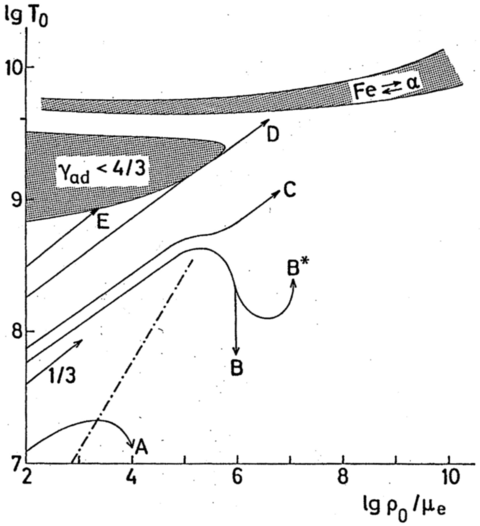Figure 2.1: Schematic evolution of the central temperature and density for different core masses. The dot-dashed line shows the boundary beyond which degeneracy is so strong (and non-relativistic) that contraction leads to a temperature decrease rather than a temperature increase. Unstable regions are hashed; that due to pair-instability is labeled γ < 4/3. Taken from KW, their Fig. 34.1.

## 3  Stability of fusion

For a non-degenerate gas, fusion is stable since a small increase in temperature and hence fusion rate leads to expansion and net cooling of the core (negative heat capacity). This can be seen from the virial theorem, or by considering homologous expansion: suppose one increases the temperature and thus pressure in some small central region. Then, the star will expand until the pressure in the central region and that required to support the rest of the star match. For homologous expansion, one has δP/P=−4δR/R, and hence in the inner region, δT/T=δP/P−δρ/ρ = −δR/R (where δρ/ρ = −3δR/R). Hence, fusion in cores is stable.
Now consider nuclear fusion in a thin shell around a core with radius r with thickness D << r (think why shells naturally will tend to become thin). The difference with the above is that now in the shell, which has mass m ≅ πr2 D, δρ = −δD/D=−(r/D)δr/r, while the weight to be carried and thus the pressure still scale as δP/P=−4δr/r. Hence, for expansion of a thin shell, the density decreases but the weight of the layers above hardly changes. Thus, the temperature will change as δT/T=δP/P−δρ/ρ = (r/D−4)δR/R, i.e., it increases for D < r/4. (See KW, §33.2 for more detail.) This instability leads to so-called thermal pulses on the asymptotic giant branch (see below).

## 4  Contraction

For optically thin emission, like in molecular cloud (or neutrino emission in a collapsing iron core), the material is roughly isothermal (P ∝ ρ, i.e., Γ = 1 < [4/3]), and hence, once unstable, collapse is inevitable.
Once the cloud becomes optically thick, further collapse is adiabatic and hydrostatic equilibrium will eventually be attained (for molecular hydrogen, Γ = [7/5], so still close to [4/3]). Further instabilities set in when hydrogen is first dissociated and then ionised.
As a star contracts on a thermal timescale (i.e., remaining in hydrostatic equilibrium), ρ ∝ R−3 and T ∝ R−1, and thus T ∝ ρ1/3. Hence, a star approaches degeneracy: Pideal ∝ ρT, PNR ∝ ρ5/3, hence lines of constant degeneracy have T ∝ ρ2/3. Denser (lower-mass) stars will become degenerate earlier, and for brown dwarfs, this happens before they become hot enough to ignite hydrogen (see Fig. ).
For radiation-dominated stars, the contraction is along the same direction, but they do not approach degeneracy: Prad ∝ T4, PER ∝ ρ4/3, hence lines of constant degeneracy have T ∝ ρ1/3. Hence, the lack of turn-over in Fig. 1 of lines starting at lower density (more massive stars).Figure 4.1: Trajectories of central conditions for low-mass (M < 0.3 M), fully convective stars as they approach the main sequence or become brown dwarfs. Note how for fully convective stars, whose internal structure in well described by a n=1.5 (Γ = [5/3]) polytrope, the trajectories follow the expected T ∝ ρ1/3 track. Tracks turn over when the conditions have become fairly degerate: Fermi energy E F/kT ≅ 4. Taken from [], their Fig. 16

## 5  Low mass stars

After the main-sequence, the cores of low-mass stars become degenerate, surrounded by a hydrogen-burning shell, and the envelopes expand, making the stars red giants.

### Shell burning

In a shell, the density and pressure drop very quickly, and hence their properties depend mostly on the properties of the core. Analogously to main-sequence stars, one can use homology arguments to show how ρ, P, T, and L scale with Mcore and Rcore. The easiest is the scaling for temperature, which, assuming an ideal gas and using hydrostatic equilibrium,
 T ∝ P ρ ∝ 1 ρ GMcoreρH Rcore2 ∝ Mcore Rcore ,
(5.1)
where we used that the scalelength H can only scale with R core assuming homology. Since fusion is generally a steep function of temperature, this means the luminosity will depend sensitively on the core properties. As the envelopes of red giants are mostly convective, and the photospheres have roughly fixed effective temperature, the radius is also a strong function of core mass.
The other scalings depends on, e.g., the temperature-dependence of the fusion process; if it is very steep, a small increase in, say, M core and thus T, will lead to a large increase in luminosity, due to which the star will expand, lowering the density. For details, see KW, §32.2.

### Helium flash

The degenerate core will be heated by its surrounding shell, and be nearly isothermal, with a slightly lower temperature in the centre due to neutrino losses. When Mcore ≅ 0.45 M, the temperature becomes hot enough for ignition, and runaway fusion starts in a shell. As this region is only moderately degenerate, convection kicks in relatively quickly, which limits the maximum temperature reached and thus avoids a dynamic event (timescales for entropy increase always remain larger than the dynamical timescale). In the end, the star settles down as a core-helium burning giant, with most of the luminosity still due to the hydrogen shell. We see such sources as red-clump stars in metal-rich populations, and horizontal-branch stars in metal-poor ones.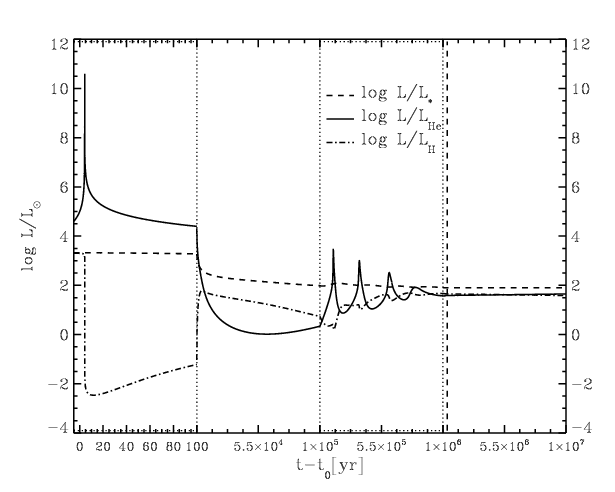Figure 5.1: Luminosities during the helium flash. The initial spike is due to the off-centre helium flash. Subsequent spikes occur when inner layers are heated up sufficiently to ignite. As the core is expanding, these reach less high temperatures and luminosities. Note how the luminosity from the hydrogen shell varies in the opposite sense of the helium luminosity, while the luminosity emitted varies much more slowly. Taken from [], their Fig. 2.Figure 5.2: Evolution of low-mass stars. One sees the core density and temperature converge for the different stars, increasing during the red giant branch up to the helium flash. The flash starts in an outer layer, at ρ ≅ 4×105 g cm3, i.e., logρ ≅ 5.6 ([]). This is close to where the 2 M track takes off and the density and temperature in the shell should evolve similarly. The cores of the lower-mass stars first expand at roughly constant degeneracy (i.e., essentially adjusting adiabatically to the decreasing weight of the overlying layers), before the core is heated such that a run-away occurs. From [], their Fig. 14.

### Double-shell burning

When two or more shells are present, they do not necessarily evolve at the same rate, leading to changing separation (in mass coordinates), and to thin shells that are geometrically unstable (see above). This underlies the thermal pulses on the asymptotic giant branch.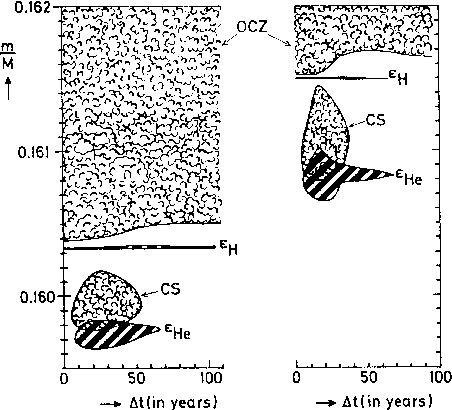Figure 5.3: Evolution of the mass shells around the two shell sources in a 5 M tar near the maximum of the first and sixth thermal pulses. From KW, Fig. 33.4.

### The Carbon flash (that doesn't happen)

Absent mass loss, for a sufficiently massive star, eventually the core density and temperature would increase sufficiently to ignite carbon. This happens as one approaches the Chandrasekhar mass, and in the core, which has a lower ignition temperature due to its very high density (note that the competition between neutrino cooling and compressional heating also makes the temperature distribution less uniform than was the case for a helium core). As one is at very high degeneracy, the run-away destroys the whole core (and surrounding envelope).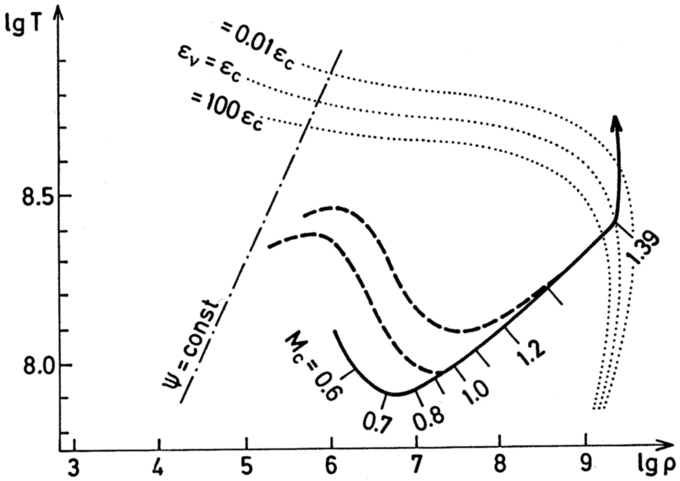Figure 5.4: Temperature and density in the CO core of a 3 M star after central helium burning. The broken lines show temperature stratifications at two instances. The core grows until carbon is ignited. In real stars, mass loss prevents the core from becoming this massive.

## 6  Ignition masses

A star with mass above the Chandrasekhar mass cannot be supported by degeneracy pressure, hence such stars continue to contract, becoming hotter, until fusion starts or some other process intervenes. Below the Chandrasekhar mass, a star heats up until it ignites fusion or becomes degenerate. The maximum temperature a star can have and still become degenerate is T=1.3×109 K (A, §6.6).
The ignition mass is the minimum mass required to reach ignition. It is  ∼ 0.08 M for hydrogen,  ∼ 0.3 M for helium,  ∼ 0.8 M for carbon, and  ∼ 1.36 M for neon []. All heavier fuels are only burnt by stars (or cores of stars) above the Chandrasekhar mass.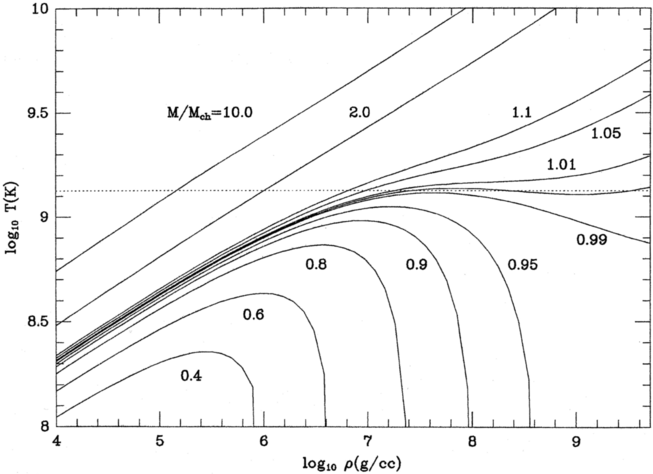Figure 6.1: Effects of contraction on temperature and density. From Arnett, his Fig. 6.3.

### Burning stages

From Arnett, his table 6.2.
• Hydrogen burning (2×107 K, (5…8)×1018 erg g1): mostly helium (via p-p or CNO; all CNO converted to N).
• Helium burning (1.5×108 K, 7×1017 erg g1): mostly carbon and oxygen (triple alpha, plus 12C(α,γ)16O, with final abundance ratio strongly dependent on rate, and on extent to which fresh helium is brought in at late stages (which would lead to more oxygen).
• Carbon burning (8×108 K, 5×1017 erg g1): mainly O, Ne, Mg, Si.
• Neon burning (1.5×109 K, 1.1×1017 erg g1): mainly O, Mg. Starts with photo-desintegration: 20Ne(γ,α)16 O, followed by 20Ne(α,γ)24Mg.
• Oxygen burning (2×109 K, 5×1017 erg g1): mainly Si, S.
• Silicon burning (3.5×109 K, (0…3)×1017 erg g1): iron-peak

## 7  Intermediate mass stars

A M\ga2 M forms a \ga0.3 M helium core and thus reaches ignition before becoming degenerate. Hence, these stars form lower-luminosity red-clump stars than initially less massive stars (for clusters with a slight spread in age, "double red clumps" have been observed; see ). Their further evolution is similar to that of lower-mass stars, though: they form CO cores and become AGB stars, with the evolution terminated by fast mass loss in the superwind. (The latter is still poorly understood theoretically; .)
For M\ga8 M (the mass is uncertain and depends on assumptions about convection, overshoot, etc.), upon helium exhaustian, a \ga0.8 M carbon core is formed, which reaches carbon ignition before becoming degenerate. Carbon fusion leaves a core composed of mostly oxygen and neon. If this core becomes degenerate before neon ignition (for core mass \la1.37 M), the star becomes a "super AGB" star.

### Electron-capture induced collapse

The further evolution of ONe cores depends on how much mass can be accreted. If less than the Chandrasekhar mass, it will become a white dwarf, while if it is more, it will reach such high densities that electron captures start, which will lead to collapse. Note that like for CO cores, neutrino cooling prevents the degenerate core from becoming hot enough to ignite the next burning stage. Unlike for CO cores, however, electron captures start to occur before the density becomes high enough to ignite neon or oxygen burning. Oxygen burning only starts as the core is already irreverably collapsing [].Figure 7.1: Evolution of central density and temperature of a ONe core that is growing in size (left), and evolution during collapse due to electron captures (right). From , his Figs 4 and 6.
In single stars, electron-capture supernovae appear unlikely: after core helium exhaustian and subsequent core contraction, the envelope expands greatly and so does the extent which is convective. For relatively low masses, this enters and removes the outer parts of the helium core. As a result, for those, the cores are left with less than the Chandrasekhar mass and will not explode, while those untouched are so massive that they do not become degenerate at all and evolve up to iron cores and core-collapse supernovae. It appears that only in binaries, with timely removal of the hydrogen envelope, electron-capture supernovae are likely [].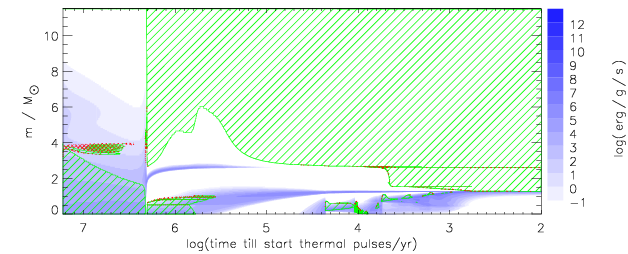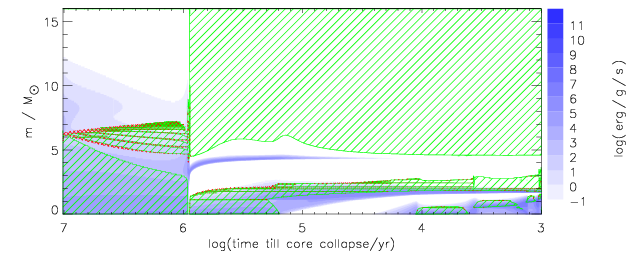Figure 7.2: Time evolution for stars with masses of 5, 11.5, and 16 M. Note the strong second dredge-up for the 11.5 M model, which greatly reduces the helium core mass, and ensures that the star ends its life as a ONe white dwarf. Taken from , their Fig. 1.Figure 7.3: Helium core mass as a function of initial mass for different stellar evolution codes, showing the effects of second dredge-up. Taken from , their Fig. 2.

## 8  Massive stars

Stars more massive than  ∼ 8 M ignite all phases of nuclear burning non-degenerately, and end with iron core collapse, leading either to a neutron star or a black hole. The details depend not just on initial mass, but also on the amount of mass loss.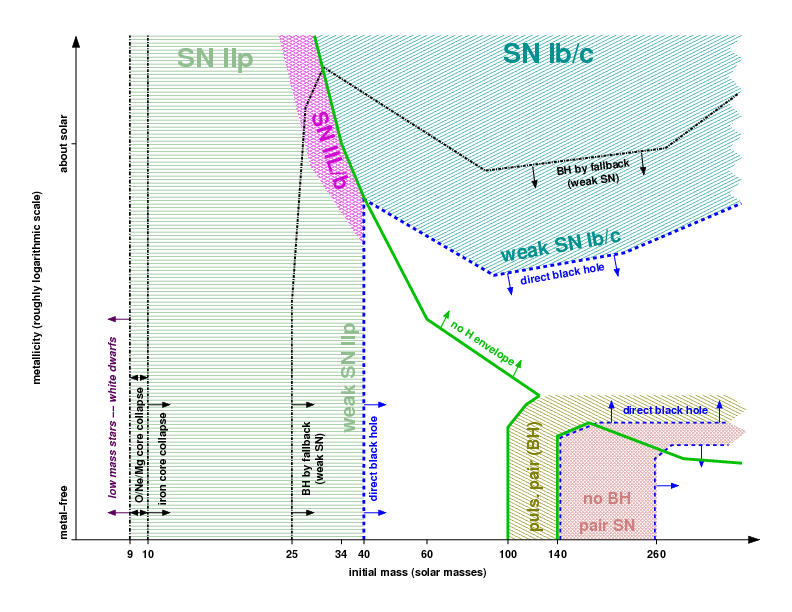Figure 8.1: Final evolution as a function of mass and metallicity - the latter influences the results mainly through the mass-loss rates. From , their Fig. 2.

## 9  Very massive stars

Stars so massive that they produce oxygen cores of \ga40 M (this may only be possible for very low-metallicity stars that experienced little mass loss), pass through the pair-instability region (see figure below and Fig. 1). There, 〈γ〉 < [4/3], and the core becomes unstable. It starts to collapse and reaches oxygen ignition. The collapse may only be halted by the time the oxygen burning timescale is less than the dynamic time, making the burning explosive. This could lead to partial or total disruption of the star, or, if the explosion is insufficiently energetic, to later disintegration and (likely) direct collapse to a black hole.Figure 9.1: Isentropic lines for a oxygen/pair plasma, with regions of interest indicated. From [], their Fig. 3.

## 10  Binary evolution

Most stars increase in radius as they evolve, often drastically. If in a binary, they may at some point overflow their Roche lobes, leading to mass transfer to the companion. If this is stable, mass transfer will be on the evolutionary timescale. If unstable, it can be on the dynamical or thermal timescale. Masses transfer ceases when the star stops trying to expand; in giants, this is when most of the envelope has been transferred, and the remainder becomes so tenuous that it shrinks. Thus, one generally is left with just the core of the star. This process, and variations on it, is responsible for most of the more interesting stars we observe.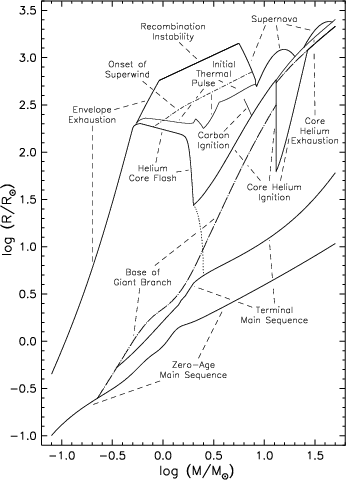Figure 10.1: Radius evolution of stars of various masses. In the left-hand panel, the one unmarked dotted line between ‘helium core flash’ and ‘core helium ignition’ marks the division between those helium cores (at lower masses) which evolve to degeneracy if stripped of their envelope, and those (at higher masses) which ignite helium non-degenerately and become helium stars. In the right-hand panel, core masses interior to the hydrogen-burning shell are indicated with solid lines, and dashed lines those interior to the helium-burning shell. Solid lines intersecting the base of the giant branch (dash-dotted curve) correspond to helium core masses of to 0.15, 0.25, 0.35, 0.5, 0.7, 1.0, 1.4, and 2.0 M; those between helium ignition and the initial thermal pulse to 0.7, 1.0, 1.4, and 2.0 M, and those beyond the initial thermal pulse to 0.7, 1.0, and 1.4 M. Dashed lines between helium ignition and initial thermal pulse correspond to carbon-oxygen core masses of 0.35, 0.5, 0.7, 1.0, and 1.4 M. Beyond the initial thermal pulse, helium and carbon-oxygen core masses converge, with the second dredge-up phase reducing helium core masses above  ∼ 0.8 M to the carbon-oxygen core. From , his Figs 1 and 2.

### Mass loss and tranfer

Consider a star that looses or transfers mass at some rate · M.Figure 10.2: ζL ≡ ∂lnRL/∂lnM2 as a function of mass ratio, with all mass transfer through a single channel: conservative (cons); isotropic wind from donor star (wind); isotropic re-emission of matter, from vicinity of `accreting' star (iso-r). (Also shown is a ring formation, indicative of mass loss from an outer Lagrange point). From , their Fig. 4.

#### Effect on orbit

The angular momentum of an orbit is given by J=(M1M2/M)√{GMa}, and thus,
 ⋅ J

J
=
 ⋅ M 1

M1
+
 ⋅ M 2

M2
1

2
 ⋅ M

M
+ 1

2
 ⋅ a

a
(10.1)
Conservative mass transfer:   · M1=−· M2, · M=0, · J=0. Thus,
 ⋅ a

a
= 2 M2−M1

M1M2

M

2
= 2(q−1)
 ⋅ M 2

M2
,
(10.2)
where q=M2/M1 is the mass ratio between the donor (star 2) and the accretor (star 1). For donors less massive than the accretor, the orbit expands upon mass transfer (remember that · M2 < 0).
Looking at the Roche lobe for a less massive donor, for which RL ≅ 0.46a(M2/M)1/3 [], one finds
 ⋅ R L

RL
=
 ⋅ a

a
+ 1

3
 ⋅ M 2

M2
= 2
q− 5

6

 ⋅ M 2

M2
,
(10.3)
showing that the Roche lobe, as expected, grows a little slower than the orbital separation. (An analysis valid for all q would use the approximation of [], RL/a ≅ 0.46q2/3/[0.6q2/3+ln(1+q1/3)].)
Spherically symmetric wind:   · M2=· M, · M1=0, · J=(· M2/M2)(M1/M)J. Hence,
 ⋅ a

a
= 2

 M1 ⋅ M

M2M
 ⋅ M

M2
+
 ⋅ M

2M

=−
 ⋅ M

M
.
(10.4)
Thus, for mass loss (· M < 0), the orbit expands.
Spherically re-emitted wind:   · M2=· M, · M1=0, · J=(· M2/M1)(M2/M)J (idea is that accretor cannot handle mass transferred to it and re-emits it as a wind). Hence,
 ⋅ a

a
= 2

 M2 ⋅ M

M1M
 ⋅ M

M2
+
 ⋅ M

2M

= 2q2−2−q

1+q
 ⋅ M

M
.
(10.5)
Hence, orbit expands for q < (1+√{17})/4=1.28 (with again a somewhat lower value for increasing Roche-lobe radius), i.e., it is less quickly unstable than for conservative mass transfer. For a more detailed analysis, see .

If the mass is lost from the outside of a star, the star becomes initially smaller, but on a hydrodynamic timescale it will partially re-expand in responds to the decreased pressure. Which effect dominates depends on the internal structure of the star. Generally, for thermal envelopes, the stars shrinks inside its Roche lobe, re-expanding only on the thermal timescale, typically to nearly its original size (especially for giants). For more detail, see . However, a complication for thermal-timescale mass transfer is that, if the secondary is substantially less massive, it cannot accrete sufficiently fast and will bloat itself. For massive stars, for M2/M1\la0.7, this leads to contact, and almost certainly further mass loss and/or a merger [,,].
Completely convective stars, or stars with deep convective layers, however, increase in size upon mass loss. For completely convective stars, which are described well by polytropes with P=Kργ with γ = [5/3] (and thus n=1.5), this follows immediately from the mass radius relation: R ∝ M−1/3 (true for constant K, i.e., for constant entropy or completely degenerate, non-relativistic gas). Comparing this to the change in Roche lobe for conservative mass transfer, one sees that stability requires that
 2 ⎛⎝ q− 5 6 ⎞⎠ < − 1 3 ⇔ q < 2 3 for    n=1.5.
(10.6)
From the work of [], it is indeed clear that for low-mass white dwarfs, dynamical instability sets in for q > [2/3]. For higher mass accretors (M1 >~0.3 M), the mass-transfer rate rapidly becomes super-Eddington, meaning some mass has to leave the system. As shown above, this implies the binary expands more and it is easier to keep mass transfer stable. [] find that, roughly, stability requires q <~0.7−0.1(M1/M).

### Common-envelope evolution

When dynamically unstable mass transfer starts, the stars enter a common envelope. This will lead to a merger unless one envelope is relatively loosely bound, e.g., if the donor is a red giant. The process is still very uncertain, and usually an energy criterion is used to decide whether or not a complete merger occurs. We write the initial orbital energy as Eorb,i=GM1M2/2ai, the final one as Eorb,f=GM1,cM2/af, and the envelope binding energy as Ee=GM1M1,e/λR1,e. Taking M1,e=M1−M1,c, a roche-lobe filling star (R1,e=RL), and assuming an efficiency α CE=Ee/(Eorb,f−Eorb,i), one finds a total shrinkage of the orbit,
 af ai = M1,c M1 ⎡⎣ 1+ 2 αCEλ ai RL M1−M1,c M2 ⎤⎦ −1
(10.7)
This shrinkage is usually very large. Tracing back the evolution of double helium white dwarfs, , found that it cannot hold for the first mass-transfer phase. They proposed an alternative description based on angular momentum loss, but this was criticised strongly by []. Overall, though, the conclusion stands that for not too extreme mass ratios, mass transfer is stabilised somehow (perhaps by irradiation driven winds; ).

### Angular momentum loss

Two stars can be driven closer by angular-momentum loss. For gravitational radiation (in a circular orbit),
 ⋅ J

J
= 32 G3

5c5
M1M2(M1+M2)

a4
,
(10.8)
implying a merger time of 1.05×107 yr(M/M)−2/3(μ/M)−1(P/1 hr)8/3, where μ = M1M2/(M1+M2) is the reduced mass, and P the orbital period. Thus, to merge within a Hubble time requires P\la0.5 d.
For binaries with low-mass stars, angular momentum can also be lost by "magnetic braking" - a solar-like wind coupled to a magnetic field. This mechanism is usually described by semi-empirical relations, which are calibrated using the rotational evolution of single stars and using population synthesis models for binaries.

### Supernova explosions

One can solve the effect of a spherically symmetric supernova explosion by consiering that, for instantaneous mass loss, the velocities of the two stars remain the same, but their mutual attraction has decreased. Thus, the instantaneous position will become the periastron of the new orbit. For given mass loss ∆M,
 rf,peri=ri
 ⇔
 af(1−e)=ai,
(10.9)
 vf,peri=v0
 ⇔
 G(M1+M2−∆M) af 1+e 1−e = G(M1+M2) ai .
(10.10)
Solving this yields
 e= ∆M M1+M2−∆M ,
(10.11)
i.e., the orbit is unbound if ∆M > [1/2](M1+M2) (as can be seen more easily from the Virial Theorem). The binary also gets a recoil kick, of
 ∆γ = M2v2−(M1−∆M)v1 M1+M2−∆M =ev1.
(10.12)
Unfortunately, the assumption that supernova explosions are spherically symmetric seems rather poor, since single radio pulsars have large space velocities, of several 100 km s1. As a result, binaries likely unbind even when relatively little mass is lost, and, conversely, may remain bound even if a large amount of mass is lost (indeed, the latter may be a requirement to understand low-mass X-ray binaries, in which neutron stars accrete from low-mass companions). There is fairly strong evidence, however, that some supernovae do not impart (large) kicks, possibly those due to electron capture (, and references therein).

### Tidal stability

For close binaries, tides will circularise the orbit. This is not possible if the mass ratio is too small. Stability requires that some angular momentum transfer from the orbit to the star changes the stellar rotation faster than the orbital one. Since J orb=(M1M2/M)√{GMa} ∝ Ω−1/3 and J star=IstarΩ ∝ Ω, stability requires that Jorb > 3Jstar. For low-mass stars, binaries with mass ratio q <~0.09 are unstable [].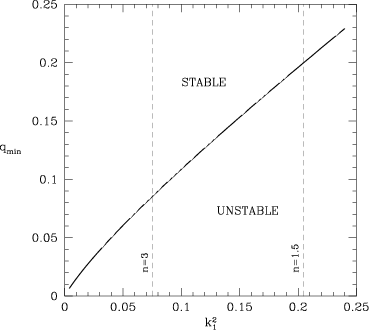Figure 10.3: Minimum mass ratio required for tidal stability as a function of gyration radius. From , his Fig. 1.

### Rapid rotation

Tidal synchronisation leads to rapid rotation. For low-mass stars, this leads to increased activity, some increase in size, and a larger stellar wind (and thus angular momentum loss; magnetic breaking).
For massive stars, rotation induces mixing []. For fairly massive stars, just brings up nitrogen. For M\ga50 M in a P\la2 d binary, centrally produced helium is efficiently mixed. As a result, these stars may burn completely to helium, and a lower-mass companion might evolve faster!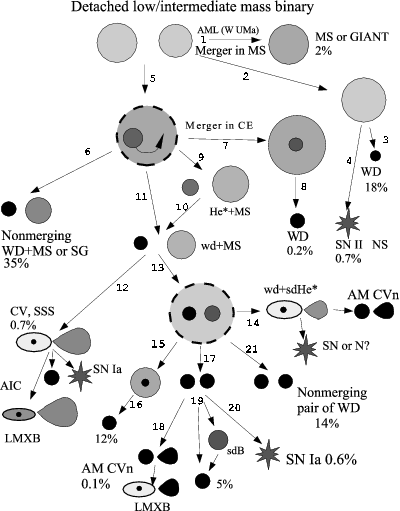Figure 10.4: Possible outcomes for low and intermediate-mass binaries. , Fig. 2 and 3.Figure 10.5: Conservative evolution of a massive binary (left) and formation of a Be X-ray binary (right). From , their Figs. 24 and 25.Figure 10.6: Further evolution of X-ray binaries with short (left) and long (right) orbital periods. From Bhattacharya & Van den Heuvel (), their Figs. 32.

## 11  Arnett's semi-analytical supernova lightcurves

We follow A96 and consider a ball of gas with initial radius R0 that is homologously expanding at constant velocity vsc, and has an initial thermal energy E0. The first law of thermodynamics can be written as,
 ⋅ E + P ⋅ V =ϵM−L,
(11.1)
where E is the total energy, V ≡ [(4π)/3]R3 is the volume, P the pressure, ϵ the energy generation rate (by radioactive decay) per unit mass, M the ejecta mass, and L the luminosity.
Assume the energy and pressure are dominated by radiation, i.e., E ≅ E, and P ≅ [1/3]E. Dividing by E on both sides and using homology, one finds,
4
 ⋅ T

T
+3
 ⋅ R

R
+ 1

3
3
 ⋅ R

R
= 4

 ⋅ T

T
+
 ⋅ R

R

= 1

τh
1

τd
,
(11.2)
where the heating timescale τh=E/ϵM and the luminosity due to cooling timescale due to diffusion of photons is τd=E/Ldiff. The latter is also given by,
 τd = κM βc R = τd,0 R0 R ,
(11.3)
where in the second equality one implicitly assumes constant opacity. For a constant density ball, β = 13.8.
The above suggests to consider the evolution of the product (TR)4. We assume its spatial (x ≡ r/R) and time dependence can be split,
 R4(t) T4(x,t) = R04 T04 ϕ(t) Ψ(x).
(11.4)
For constant density ρ = M/[4/3]πR3 and constant opacity κ,
 Ψ(x) = sin(πx) πx .
(11.5)
In terms of these functions, the thermal energy can be written as,
 E = ⌠⌡ R 0 aT(r,t)44πr2  dr = 4πR3 aT(0,t)4 ⌠⌡ 1 0 Ψ(x)x2 dx = 4 π R03 aT04 R0 R ϕ(t) = E0 R0 R ϕ(t)
(11.6)
where we used that ∫01Ψ(x)x2 dx=1/π2. The factor R0/R accounts for adiabatic expansion and ϕ(t) for radiation loss and radioactive heating. Given this, the luminosity is given by
L = E

τd
=
 E0 R0 R ϕ(t)

 τd,0 R0 R
= L0ϕ(t).
(11.7)
Supposing the initial thermal energy is of order the kinetic energy, i.e., E0 ≅ [1/2]Mvsc2, the initial lumonosity L0=E0d,0 ∝ vsc2R/κ is independent of mass, but proportional to radius. Faster ejections (larger energy) from larger stars (faster diffusion) give more luminous transients.

### Diffusion and heating

With just diffusion and heating, one has
 d dt (TR)4

(TR)4
=
 ⋅ ϕ

ϕ
= 1

τh
1

τd
⇔
 ⋅ ϕ

ϕ
=
ϵ/ϵ0

τh,0ϕ
1

τd,0

R

R0
,
(11.8)
where we tried to write in terms of ratios on the right-hand side, with ϵ/ϵ0 capturing the time dependence of the heating process (and where we again implicitly assumed constant opacity).
Ignoring heating, an analytic solution is possible. Using that τdd,0(R0/R)=τd/(1+vsct/R0), and defining an expansion timescale τe=R/vsc, one finds
 ϕ = exp ⎛⎝ − t τd,0 − t2 2τeτd,0 ⎞⎠ .
(11.9)
Generally, τd,0 >> τe, and thus for t > τe, the lightcurve is essentially a Gaussian, with a timescale that is the geometric mean of the expansion and diffusion times scales, τexp=√{τhτ d,0} ∝ √{κM/vsc}. Slower, more massive ejections lead to longer transients.
Including heating, the integration needs to be done numerically. However, generally, one expects maximum to occur when · ϕ=0, i.e., when 1/τh=1/τd (of course, if heating is too small, this maximum after explosion never happens). From their definitions, the timescales match when L=ϵM. Thus, maximum luminosity gives a measure of the total amount of radioactive decay - "Arnett's rule." (This will be an underestimate if the opacity is decreasing with time - or if this is happening effectively due to recombination.)

### Including recombination

At some temperature Ti, material will recombine and become essentially transparent. If this happens inside the cloud, then this will effectively be at optical depth zero, and the photosphere would be at Teff4 ≅ 2Ti4. As more matter recombines, the photosphere will move in, with recombination and advection ("freed" radiation) giving additional sources of luminosity. At this time, one will have,
(11.10)
where Ri=xiR is the radius of the recombination front, and where we used the subscript "min" as a reminder that the luminosity cannot be lower than this value for this radius.
The luminosity due to recombination is
 Lrec = −4πRi2 ⋅ R i ρQ = −3xi2 ⋅ x i 4π 3 R3ρQ = −3xi2 ⋅ x i MQ,
(11.11)
where Q is the energy release per unit mass due to recombination.
For the advection and diffusion terms, the results depend on whether the front moves slow or fast compared to the time to adjust the overall temperature structure. Generally, though, L diff = Ed and,
 Ladv = − ⋅ x i ∂E ∂xi
(11.12)
but the total thermal energy E and diffusion timescale τd may now depend on xi. In consequence, not only the differential equation for ϕ has to be solved, but also one for the recombination front position xi. The latter can be derived from the constraint that the additional luminosity Lrec+Ladv has to match the excess luminosity L min−Ldiff, or
 − ⋅ x i ⎡⎣ 3xi2MQ+ ∂E ∂xi ⎤⎦ = 4πR2 xi2 2σTi4 − E τd .
(11.13)
Below, we will also use the timescale on which the initial energy would be radiated at an effective temperature of 21/4Ti,
τi,0 E0

Lmin,0
=
 4 π R03aT04

 4πR022 ac 4 Ti4
= 4R0

π2 c
T04

2Ti4
.
(11.14)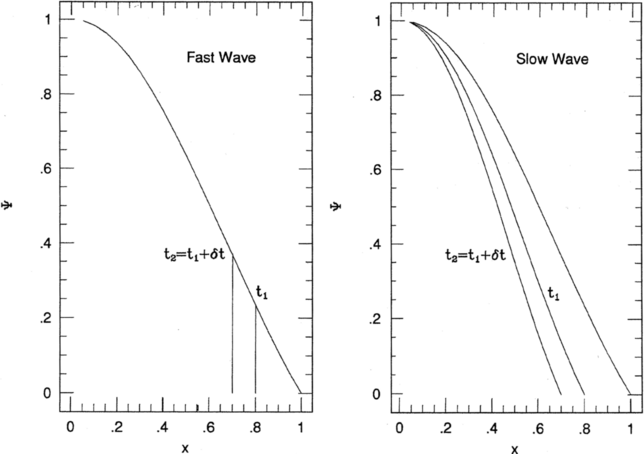Figure 11.1: Fast and slow approximation to a recombination wave. From A96, his Fig. 13.7.

#### Slow recombination front

If the recombination front moves slowly, photon diffusion inside it will ensure the temperature structure adjusts to its new outer boundary, Ri=xiR, with the same spatial structure ([T(x)/T(0)]4=Ψ(x)). Thus, the total thermal energy will be
 E = 4πR3 aT(0,t)4 ⌠⌡ xi 0 Ψ(x/xi)x2 dx = E0 R0 R ϕ(t)xi3,
(11.15)
where ϕ(t) accounts for changes in central properties due to the recombination wave and associated energy loss. Given this, the advection luminosity is given by,
 Ladv = − ⋅ x i ∂E ∂xi = −3xi2 ⋅ x i E0 R0 R ϕ(t).
(11.16)
Since the size is decreasing, the luminosity due to photon diffusion also changes, becoming
 Ldiff = E τd = E0 τd,0 ϕ(t)xi,
(11.17)
where we used that τdd,0(R0/R)xi2, with the dependence on xi2 reflecting the dependence of τd on M/R (for constant density the mass enclosed within the recombination front scales with xi3). The differential equations to be solved thus become,
 ⋅ ϕ

ϕ
 =
 ϵM E0ϕxi3 R R0 − 1 τd,0xi2 R R0 ,
(11.18)
 −3xi2 ⋅ x i ⎡⎣ MQ+E0 R0 R ϕ ⎤⎦
 =
 4πR2 xi2 2σTi4 − E0 τd,0 ϕxi.
(11.19)
Simplifying,
 ⋅ ϕ

ϕ
 =
 ⎡⎣ ϵ/ϵ0 τh,0ϕxi3 − 1 τd,0xi2 ⎤⎦ R R0
(11.20)
 −3xi2 ⋅ x i
 =
 xi2 τi,0 ⎛⎝ R R0 ⎞⎠ 2 − ϕxi τd,0

 MQ E0 + R0 R ϕ
(11.21)

### Fast recombination front

For a fast-moving recombination front, the temperature structure inside will not react to the fact that the outer parts are being chopped off. The total thermal energy inside the recombination wave is,
 Ex < xi = 4πR3 aT(0,t)4 ⌠⌡ xi 0 Ψ(x)x2 dx = E0 R0 R ϕ(t) π2 ⌠⌡ xi 0 Ψ(x)x2 dx,
(11.22)
and thus the advection luminosity is given by,
 Ladv = − ⋅ x i ∂Ex < xi ∂xi = −3xi2 ⋅ x i π2 3 Ψ(xi) E0 R0 R ϕ(t).
(11.23)
The luminosity due to photon diffusion from the inside now changes only because we are evaluating it at a different position, becoming
 Ldiff = L0diff |−x2∂Ψ/∂x|xi |−x2∂Ψ/∂x|1 = E0 τd,0 ϕ(t) ⎢⎢ −x2 ∂Ψ ∂x ⎢⎢ xi = E0 τd,0 ϕ(t)π2I(xi).
(11.24)
where L0diff is the diffusion luminosity we would obtain ignoring the recombination wave, and where we have used that [−x2∂Ψ/∂x]xi = (1/π)sin(πxi)−xicos(πxi) = π2I(xi) (where π2I(xi)=π20xiΨ(x)x2dx is the normalised integral).
The differential equations to be solved now become,
 ⋅ ϕ

ϕ
 =
 ϵM E0ϕπ2I(xi) R R0 − 1 τd,0 R R0 ,
(11.25)
 −3xi2 ⋅ x i ⎡⎣ MQ+E0 R0 R ϕ π2 3 Ψ(xi) ⎤⎦
 =
 4πR2 xi2 2σTi4 − E0 τd,0 ϕπ2I(xi).
(11.26)
Simplifying,
 ⋅ ϕ

ϕ
 =
 ⎡⎣ ϵ/ϵ0 τh,0π2I(xi) − 1 τd,0 ⎤⎦ R R0
(11.27)
 −3xi2 ⋅ x i
 =
 xi2 τi,0 ⎛⎝ R R0 ⎞⎠ 2 − ϕπ2I(xi) τd,0

 MQ E0 + R0 R ϕ π2 3 Ψ(xi)
(11.28)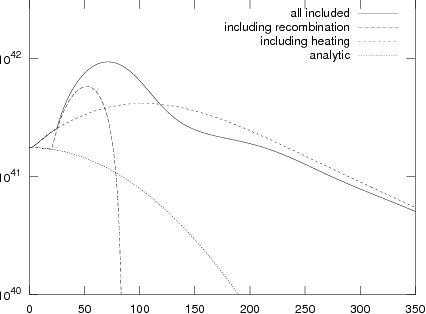Figure 11.2: Comparison of explosions with and without recombination and heating by radioactive decay. Note that I could not reproduce all curves in A96 in detail, in particular not for the "slow" case. Still, the general trends are clear and should be correct.Figure 11.3: Semi-analytic lightcurves for supernovae with varying properties. Those not varied are held fixed at those inferred for SN 1987A by A96 (his Table 13.2): Mej=15 M, E SN=1.7×1051 erg, R0=3×1012 cm, κ = 0.2 cm2 g1, MNi=0.075 M, Tion=4500 K, Q ion=13.6 eV nucleon1. Ignored is losses of gamma rays, and hence the luminosity at late times is overestimated.

File translated from TEX by TTH, version 4.03.
On 27 Mar 2013, 12:46.